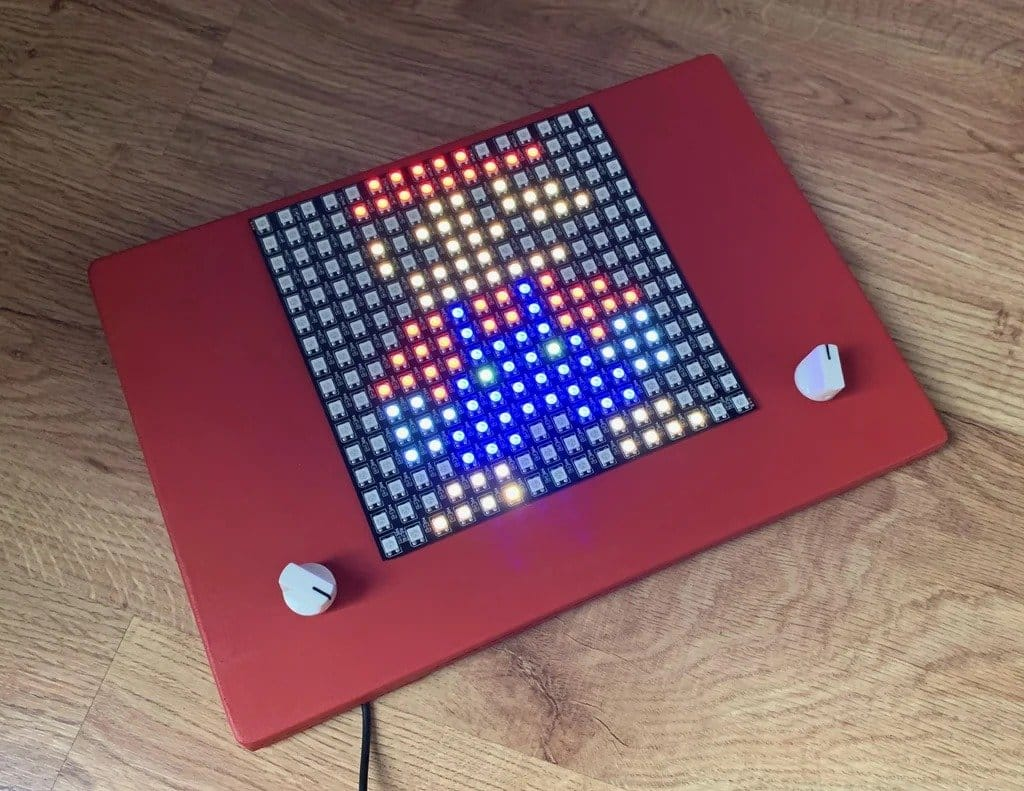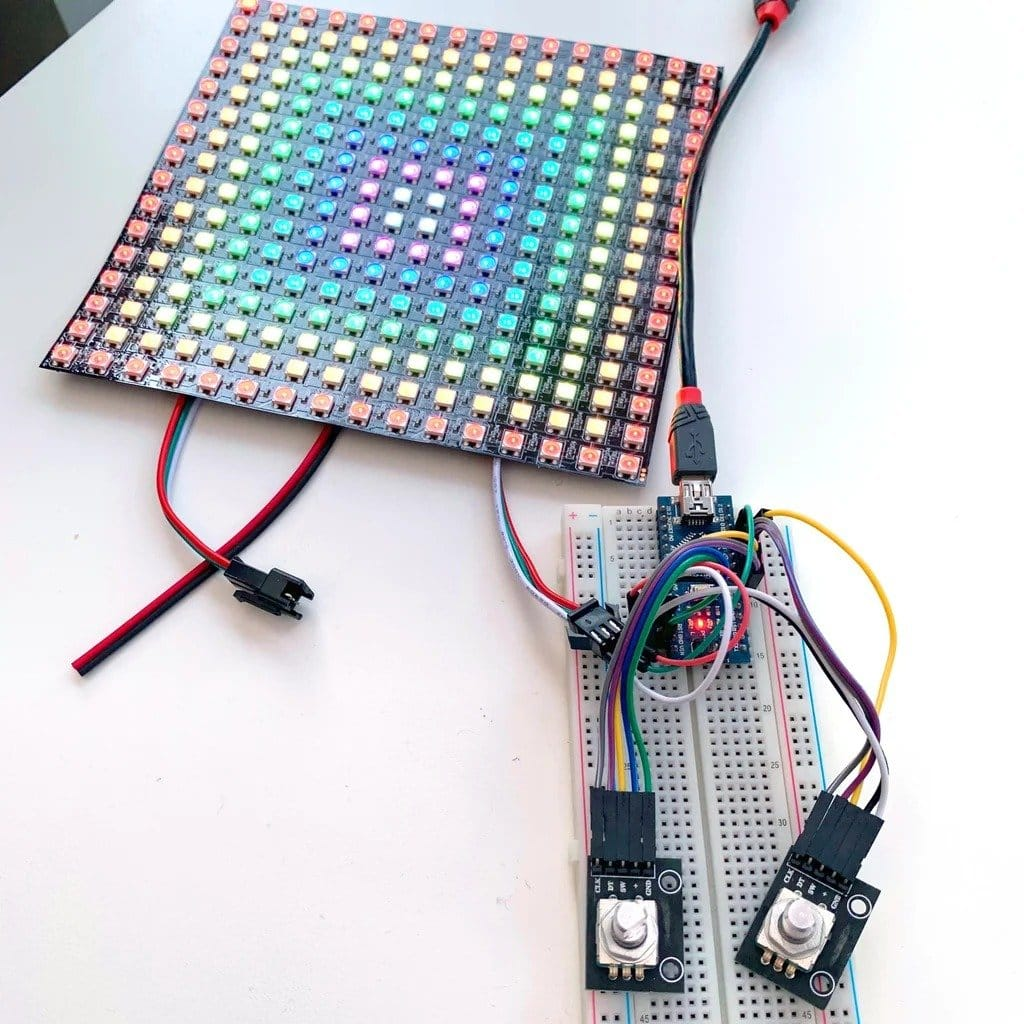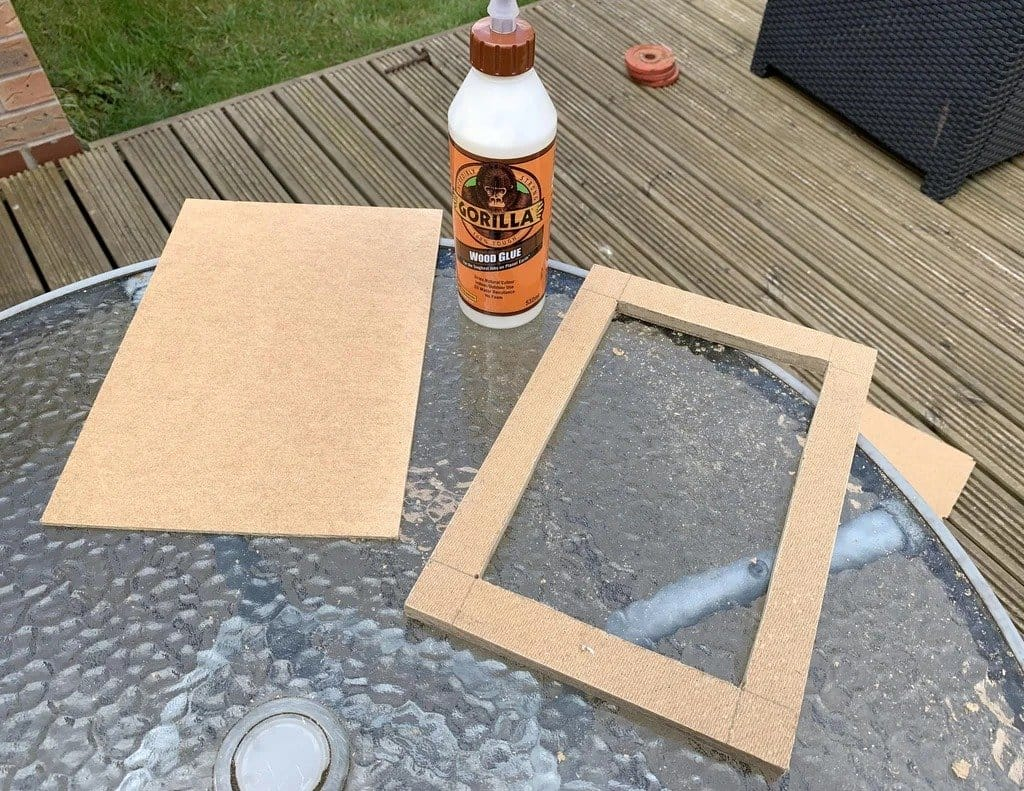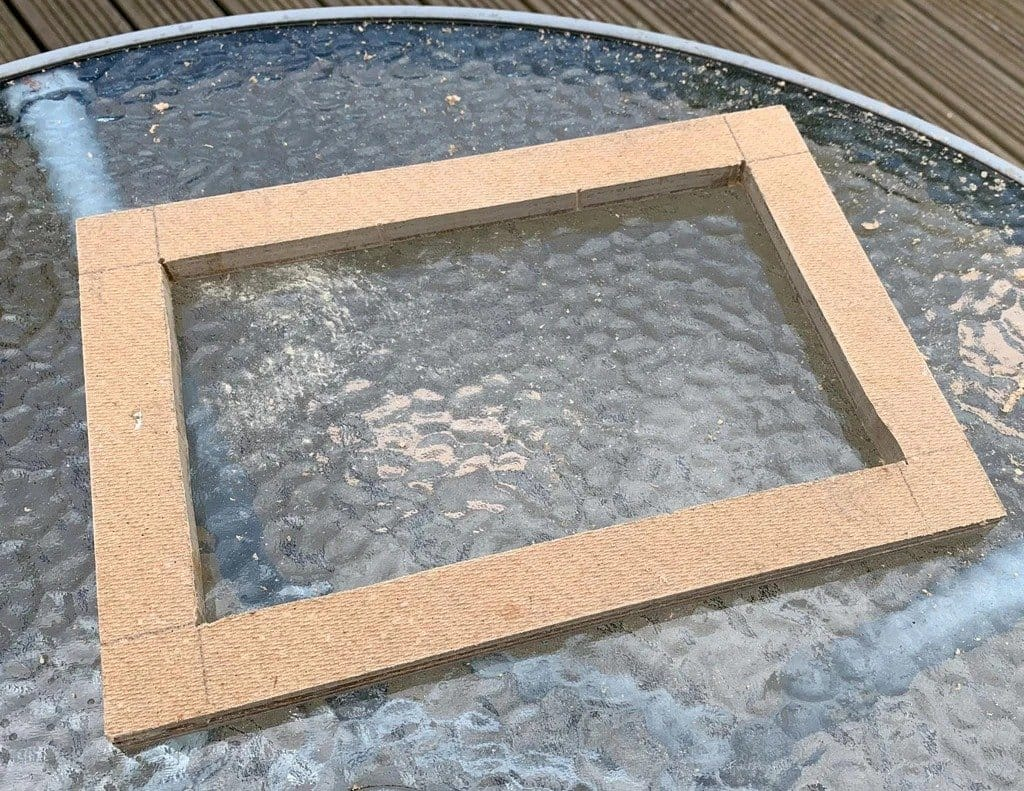DIY

# LED board with addressable LEDsPixel art can be created with this LED board. By turning the two encoders, the horizontal and vertical position of the cursor is controlled. To change the color, the encoder must be pressed. All of this is controlled by the Arduino Nano.
Tools and materials: -Arduino Nano; -Two rotary encoders with a push switch; -Two knobs for encoders; -LED matrix WS2812b 16 x 1;
-Breadboard; -Jumpers -15 pcs; -Power supply;
-MDF;
-PVA glue;
-Red paint;
-Glue gun; Step one: circuit
First, the master checked the circuit using a breadboard. The LED matrix needs to use an additional power supply, although the master powered it directly to the Arduino.Step two: code
For the matrix to work, you will need to install the FastLED and LEDMatrix libraries.
Not all LED matrix panels have the same configuration. The LEDMatrix library includes various configurations, you need to experiment with lines 14, 15 and 16.

` `//RGB LED Etch-A-Sketch //Author & # 58; John-Lee Langford //Website & # 58; www.mr.langford.co //Includes #include & lt; FastLED.h & gt; //https & # 58; //github.com/FastLED/FastLED #include & lt; LEDMatrix.h & gt; //https & # 58; //github.com/AaronLiddiment/LEDMatrix //Change the next 6 defines to match your matrix type and size #define LED_PIN 10 #define COLOR_ORDER GRB #define CHIPSET WS2812 #define MATRIX_WIDTH -16 //Set this negative if physical led 0 is opposite to where you want logical 0 #define MATRIX_HEIGHT -16 //Set this negative if physical led 0 is opposite to where you want logical 0 #define MATRIX_TYPE VERTICAL_ZIGZAG_MATRIX //See top of LEDMatrix.h for matrix wiring types cLEDMatrix & lt; MATRIX_WIDTH, MATRIX_HEIGHT, MATRIX_TYPE & gt; leds; //Rotary A - Horizontal movement int horizontalA = 9; //DT int horizontalB = 8; //CLK int buttonA = 7; //Button int btnA; //Button A value int horizontalALast; int horizontal, horizontalCursor = 0; unsigned char encoder_horizontalA; unsigned char encoder_horizontalB; unsigned char encoder_horizontalA_prev = 0; //Rotary B - Vertical movement int verticalA = 6; //DT int verticalB = 5; //CLK int buttonB = 4; //Button int btnB; //Button B value int verticalALast; int vertical, verticalCursor = 0; unsigned char encoder_verticalA; unsigned char encoder_verticalB; unsigned char encoder_verticalA_prev = 0; //Common unsigned long currentTime; unsigned long loopTime; bool moved = false; //Colors const int numberColours = 9; //Total number of colors available unsigned long colors & # 91; numberColours & # 93; & # 91; 2 & # 93; = & # 123; & # 123; 0xFF0000, 0x330000}, & # 123; 0xFF6600, 0x331100}, & # 123; 0xFFFF00, 0x333300}, & # 123; 0x00FF00, 0x003300}, & # 123; 0x00FFFF, 0x001111}, & # 123; 0x0000FF , 0x000033}, & # 123; 0xFF00FF, 0x330033}, & # 123; 0xFFFFFF, 0x111111}, & # 123; 0x111111, 0x000000}}; int currentColour = 0; //Set to first color in array void setup () & # 123; FastLED.addLeds & lt; CHIPSET, LED_PIN, COLOR_ORDER & gt; (leds & # 91; 0 & # 93 ;, leds.Size ()); FastLED.setBrightness (255); //Clear the display in a pretty way int colourBounce = 1; for (int i = 0; i & lt; 16; i ++) & # 123; leds.DrawLine (0, i, 15, i, colors & # 91; currentColour & # 93; & # 91; 0 & # 93;); leds.DrawLine (i, 0, i, 15, colors & # 91; currentColour & # 93; & # 91; 0 & # 93;); FastLED.show (); delay (25); leds.DrawLine (0, i, 15, i, CRGB (0, 0, 0)); leds.DrawLine (i, 0, i, 15, CRGB (0, 0, 0)); currentColour = currentColour + colourBounce; if (i == 7) & # 123; colourBounce = -1; }} FastLED.clear (true); //Rotary A - Horizontal pinMode (horizontalA, INPUT); pinMode (horizontalB, INPUT); pinMode (buttonA, INPUT); horizontalALast = digitalRead (horizontalA); //Rotary B - Vertical pinMode (verticalA, INPUT); pinMode (verticalB, INPUT); pinMode (buttonB, INPUT); verticalALast = digitalRead (verticalA); //Common currentTime = millis (); loopTime = currentTime; } void loop () & # 123; //get the current elapsed time currentTime = millis (); if (currentTime & gt; = (loopTime + 5)) & # 123; //Read encoder pins encoder_horizontalA = digitalRead (horizontalA); encoder_horizontalB = digitalRead (horizontalB); encoder_verticalA = digitalRead (verticalA); encoder_verticalB = digitalRead (verticalB); //Check for button presses btnA = digitalRead (buttonA); btnB = digitalRead (buttonB); if (btnA == 0) & # 123; currentColour ++; if (currentColour & gt; (numberColours - 1)) & # 123; currentColour = 0; } delay (500); } if (btnB == 0) & # 123; currentColour--; if (currentColour & lt; 0) & # 123; currentColour = numberColours - 1; } delay (500); } //Encoder A if ((! Encoder_horizontalA) & amp; & amp; (encoder_horizontalA_prev)) & # 123; //A has gone from high to low if (encoder_horizontalB) & # 123; //B is high so counter-clockwise if (horizontalCursor & gt; 0) & # 123; horizontalCursor -; moved = true; }} else & # 123; //B is low so clockwise if (horizontalCursor & lt; 15) & # 123; horizontalCursor ++; moved = true; }}} encoder_horizontalA_prev = encoder_horizontalA; //Store value of A for next time //Encoder B if ((! Encoder_verticalA) & amp; & amp; (encoder_verticalA_prev)) & # 123; //C has gone from high to low if (encoder_verticalB) & # 123; //B is high so counter-clockwise if (verticalCursor & lt; 15) & # 123; verticalCursor ++; moved = true; }} else & # 123; //D is low so clockwise if (verticalCursor & gt; 0) & # 123; verticalCursor -; moved = true; }}} encoder_verticalA_prev = encoder_verticalA; //Store value of C for next time loopTime = currentTime; //Updates loopTime leds (horizontalCursor, verticalCursor) = colors & # 91; currentColour & # 93; & # 91; 0 & # 93 ;; //Move the LED if (moved) & # 123; leds (horizontal, vertical) = colors & # 91; currentColour & # 93; & # 91; 1 & # 93 ;; horizontal = horizontalCursor; vertical = verticalCursor; moved = false; } FastLED.show (); }} ` `

Step Three: Frame
For the manufacture of the frame, the master used 6 mm MDF sheets. First, he cut four A4 sheets. Then, stepping back from the edge 2.5 cm, cut out the middle of three blanks. These three sheets, when glued together, should provide enough depth to accommodate the Arduino and other components.
Then four MDF sheets were glued with PVA glue. When the PVA was completely hardened, the edges were sanded.
Drilled holes in the front of the case. Two, at the edges, for encoders. Three central, for wires.
I painted the frame red.Step four: assembly
To make the device as thin as possible, the master used thin strips of perforated board (1 x 4) for power supply.
The encoders are screwed in with the supplied nuts, the rest of the parts are fixed with hot melt glue.Step five: control
The matrix is ​​controlled as follows.
When turning left encoder, the cursor moves left and right. Pressing the encoder moves the cursor forward.
Turning the right encoder moves the cursor up and down. Clicking on the encoder moves the cursor backward.
When the cursor moves, the selected color remains at the previous “pixel.”
The cursor is displayed brighter than other pixels, so users can see where it is.Source:

usamodelkina.ru